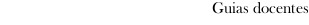Educational guide 2019_20Escola de Enxeñería Industrial
 Degree in Industrial Technologies EngineeringSubjectsBasics of circuit analysis and electrical machinesContents
 Topic Sub-topic SUBJECT 1. INTRODUCTION And AXIOMS 1.1 Magnitudes and units. 1.2 References of polarity. 1.3 Concept of electrical circuit. 1.4 Axioms of Kirchhoff. SUBJECT 2. ANALYSIS OF LINEAR CIRCUITS RESISTIVES 2.1 Ideal Elements: definition, representation and mathematical model. 2.2 Models of real sources. 2.3 Equivalent Dipoles: conversion of sources. 2.4 Association of resistors: concept of voltage divider and current divider. 2.5 Association of sources and resistors. 2.6 Topological Concepts: knot, branch, bow and mesh. 2.7 Number and election of circular and nodal equations linearly independent. 2.8 Analyses by meshes and knots of circuits with resistors. 2.9 Topological Transformations. 2.10 Power and energy in resistors, ideal sources and real sources. 2.11 Fundamental theorems. SUBJECT 3. ANALYSIS OF CIRCUITS WITH ELEMENTS THAT STORE ENERGY 3.1 ideal Condenser: definition, representation and mathematical model. 3.2 magnetic Circuits: units, magnetic flow, strength magnetomotive and reluctance. 3.3 ideal Coil: definition, representation and mathematical model. 3.4 Association series and parallel of coils and capacitors. 3.5 Circuits with elements that store energy. Circuits RL, RC and RLC. SUBJECT 4. ANALYSIS OF CIRCUITS IN SINUSOIDAL STEADY-STATE REGIME 4.1 Forms of periodic wave and values associated: sinusoidal wave. 4.2 Determination of the sinusoidal steady-state regime. 4.3 Response of the basic passive elements to sinusoidal excitations: concept of impedance and complex admittance. 4.4 Law of Ohm and axioms of Kirchhoff in sinusoidal steady-state regime. 4.5 Association of elements. 4.6 Analyses by knots and by meshes of circuits in sinusoidal steady-state regime. 4.7 Power and energy in sinusoidal steady-state regime. Instantaneous power, half or active power and energy in the passive elements: coils, capacitors, resistances and complex impedances. 4.8 Power and energy in the dipoles. Apparent power, reactive power and complex power. 4.9 Theorem of conservation of the complex power (theorem of Boucherot). 4.10 The power factor and his importance in the electrical systems. Correction of the power factor. 4.11 Measurement of the active and reactive power: wattmeters and varmeters. 4.12 Fundamental Theorems in sinusoidal steady-state regime. SUBJECT 5: MAGNETIC ADJUSTMENTS 5.1 Magnetic joined up coils: definitions, equations of flows, own and mutual inductances. Representations and mathematical models. 5.2 Analyses by meshes of circuits of alternating current with coils joined up. SUBJECT 6: BALANCED THREE-PHASE SYSTEMS 6.1 Introduction. Three-phase voltage system. Sequence of phases. 6.2 Generators and three-phase loads: star and triangle connections. Voltages and currents. 6.3 Equivalent transformations star-triangle. 6.4 Analyses of balanced three-phase systems. Equivalent single-phase circuit. 6.5 Power in balanced three-phase systems. Compensation of the power factor. SUBJECT 7. ELECTRICAL MACHINES 7.1 Transformer and autotransformers. 7.2 Rotational electrical machines: synchronous machine, asynchronous machine and DC machines. PRACTICES 1. Use of lab equipments. 2. Measures in resistive circuits. 3. Introduction to the analysis and simulation of circuits by means of Matlab. 4. Determination of a linear model of a real coil with core of air. Real coil with core of iron. Cycle of magnetic hysteresis. 5. Simulation of transient regime by means of Matlab. 6. Measures of active and reactive power in monophase systems. Compensation of the power factor.
 Universidade de Vigo            | Reitoría | Campus Universitario | C.P. 36.310 Vigo (Pontevedra) | Spain | Tlf: +34 986 812 000